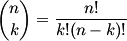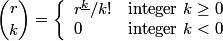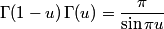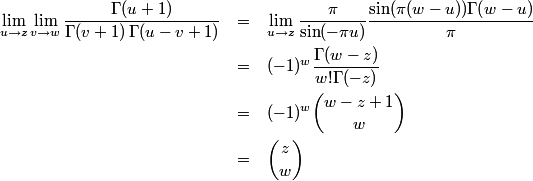# Binomial coefficients

This page gives three definitions of binomial coefficients in order of increasing generality.

## Definition 1: most basic

The most common definition of binomial coefficients is not the most useful or the most general. Most sources define the binomial coefficient (n, k) asfor where n is a positive integer and 0 ≤ kn. Call this Definition 1. It turns out to be useful to replace the above definition with one that allows n to be any real number and allows k to be any integer.

## Definition 2: more general

The book Concrete Mathematics defines binomial coefficients as follows. First, define the kth falling power of r asThe kth falling power of r multiplies starts with r and multiplies together k numbers, each being one less than its predecessor. Given this notation, define the binomial coefficients asCall this Definition 2. Note the use of “r” rather than “n” to remind us that the top number can be any real number, not necessarily an integer. When Definition 1 and Definition 2 both hold, they give the same result. When only Definition 2 holds, the value can no longer be interpreted combinatorially. That is, it doesn’t make sense to think of “r things taken k at a time” or “choosing k things from a set of r items” when r is not an integer or when k is negative.

So why the new definition? Here are four reasons.

1. Many theorems can be stated more simply in terms of Definition 2. There is less need to include qualifications in theorem statements. For example, the cases kn and k > n can often be handled uniformly.
2. Definition 2 turns out to be the correct generalization to allow many theorems to extend to more general arguments. For example, the binomial theorem extends to general exponents when binomial coefficients are defined this way.
3. Sums can often be expressed as summations over all integers; the terms that you’d otherwise need to explicitly exclude turn out to be zero. This simplifies formulas and reduces errors.
4. The binomial coefficient (r, k) is a kth degree polynomial in r, which turns out to be very handy in proofs. It’s often possible to show that two binomial coefficients are equal by showing that the corresponding polynomials agree at k+1 points and hence must be equal everywhere.

The choose(r, k) function in R implements this definition.

## Definition 3: most general

Definition 2 is adequate for many applications, but there is a still more general definition. For any complex numbers z and w, the binomial coefficient (z, w) can be defined as follows.Call this Definition 3. Note that the order of the limits is important. More on that below.

If we define z! for a complex number to be Γ(z+1) then Definition 3 looks like Definition 1, except for the limits. What’s up with the limits? They are necessary to properly handle the cases where z or w are singularities of the Γ function. It is not obvious that Definition 3 gives the same values as Definition 2 when both definitions apply, but we will show that this is the case.

The singularities of the Γ function occur at 0, -1, -2, etc. and so the limit in Definition 3 is non-trivial when z or w are negative integers. If w is a negative integer, the limit is zero because the denominator goes to ∞. We do not need to be concerned about whether z is also a negative integer because the limit in v is evaluated before the limit in u.

If w is not an integer but z is a negative integer, the binomial coefficient is infinite because the denominator is bounded but the numerator goes to ∞. This does not contradict Definition 2 because that definition only allows integer values of w.

Suppose w is a non-negative integer. We show that Definition 3 gives the same value as Definition 2. First we apply the reflection identityto Γ(u+1) and Γ(uv+1). ThenThe Binomial[r, k] function in Mathematica implements this definition.